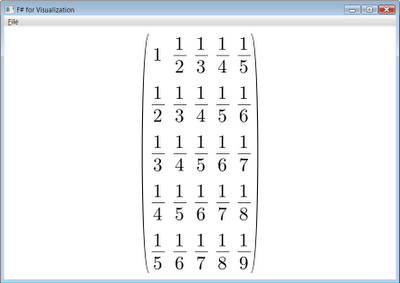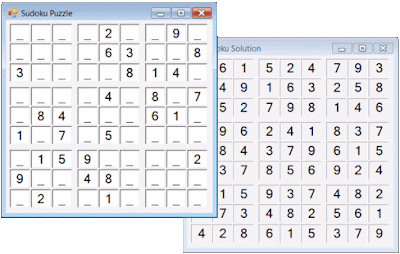## Wednesday, 17 June 2009

### Least squares: a case study in optimization

The F#.NET Journal just published an article about curve fitting:

"The challenge of finding the least squares best fit of a linear sum of functions is a common problem in regression. Linear algebra provides a powerful and general solution by expressing this problem in terms of matrices and then computing the QR decomposition in order to solve the matrix equation. This article describes a remarkably simple implementation of QR decomposition in F# before developing progressively more optimized implementations using the guidance of performance profiles and without sacrificing generality. In particular, heavy use of the F# keyword "inline" is made in order to express efficient reusable numerical methods..."

## Friday, 12 June 2009

### F# for Numerics 0.2.0.2 released

A new version of our F# for Numerics library for F# has been released. This version includes:

• Eigenvalues and eigenvectors of real symmetric matrices (the LinearAlgebra.Float.symmetric_eigen and LinearAlgebra.Float32.symmetric_eigen functions).
• Singular Value Decomposition of real matrices (the LinearAlgebra.Float.svd and LinearAlgebra.Float32.svd functions).
• Complex Cholesky decomposition of positive definite Hermitian matrices (the LinearAlgebra.Complex.cholesky function).
• Real and complex Log Gamma function (the Special.gammaln and Special.Complex.gammaln functions).
• Real and complex Beta function (the Special.beta and Special.Complex.beta functions).

The HTML documentation for this product is freely available on-line.

## Wednesday, 10 June 2009

### F# for Visualization 0.4.0.0 released

F# for Visualization version 0.4.0.0 has just been released. This version includes a variety of enhancements.

Perhaps the most important enhancement is the introduction of dynamically typed functions scene and Typeset.math which try to build vector scenes and typeset mathematical layouts, respectively, from values of any type. The View function can be used to visualize any value of any type. For example, the following defines and then visualizes a matrix of rational numbers:

```> let A =
Matrix.Generic.of_seq
[ for i in 0N..4N ->
[ for j in 0N..4N ->
1N / (i + j + 1N) ] ];;
val A : Matrix<BigNum> = matrix [[1N; 1/2N; 1/3N; 1/4N; 1/5N]
[1/2N; 1/3N; 1/4N; 1/5N; 1/6N]
[1/3N; 1/4N; 1/5N; 1/6N; 1/7N]
[1/4N; 1/5N; 1/6N; 1/7N; 1/8N]
[1/5N; 1/6N; 1/7N; 1/8N; 1/9N]]
> View A;;```This makes it a lot easier to see what is going on during matrix computations!

Plots may now be augmented with an "epilog". For example, the following creates a graph of the sinc function:

`let g = Plot([Function sinc], (-12., 12.), (-0.3, 1.1))`Accurately typeset axis labels may be added to create a professional-quality plot:

`g.Labels <- (Char 'x', Row[Text "sinc"; math "(x)"])`Finally, the first positive root of this function may be highlighted by adding a single data point and a label describing the exact coordinate:

```let pi = System.Math.PI
g.Data <- [Function sinc; Data[pi, 0.]]
g.Epilog <- Label(scene(Row[math "("; Greek.pi; math ", 0)"]),
0.003, Point(System.Math.PI, 0.), Vector(1.2, 1.2))```Axis and grid styles and the font sizes of ticks are now configurable.

Finally, the DLL is 30% smaller than before!

## Monday, 1 June 2009

### Sudoku Solver demo from the F#.NET Journal

The F#.NET Journal just made another downloadable demo freely available. This demo is from the article Designing and implementing a Sudoku Solver (30th June 2007).The entire program, developed and explained in the article, including logic solver and programmatic GUI is under 150 lines of F# code!

### Visualizing linear algebra: Singular Value Decomposition

The F#.NET Journal just published an article about the interactive visualization of linear algebra:

"Singular Value Decomposition (SVD) is an important algorithm from linear algebra that decomposes a matrix into the product of three simpler matrices. The resulting decomposition has several applications. This article describes the design and implementation of an F# program that computes the SVD of an image and uses it to create a lossy compression algorithm with controllable quality. This involves numerical methods for computing the eigenvalues of real symmetric matrices and interactive visualization of the result as a standalone Windows Presentation Foundation application that uses the Task Parallel Library to leverage multicores..."